Djilali Benayat

## Graphing on the Riemann SphereNBCDFPDF

We give a procedure to plot parametric curves on the sphere whose advantages over classical graphs in the Cartesian plane are obvious whenever the graph involves infinite domains or infinite branches.

### Introduction

Graphing a curve in the Cartesian plane can be done only in a restricted “window”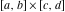. If the function to be plotted has a large domain or range, it is practically impossible to get a global view of the curve. This makes it difficult to understand the asymptotic behavior of complicated curves with various kinds of infinities. Furthermore, for most functions (e.g., polynomials of degree greater than four), graphing in a large window loses important details, while graphing in a small window loses the global features.

The remedy is to compactify the plane and represent graphs on the Riemann sphere. The usual method is to map the plane graph to the sphere using the inverse stereographic projection. We prefer a slightly modified version: we smoothly wrap the plane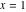on the sphere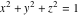using the inverse stereographic projection from the pole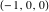. The origin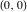maps to the blue point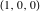on the sphere, and the point at infinity maps to the red point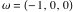.

### Benefits of the Method

#### Asymptotic Behavior

Although the point at infinity cannot be reached, the mapping gives points so close tothat it is as if we had reached it. As an illustration, here are the graphs of a polar curve first in the plane (with asymptotes) and then on the sphere.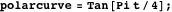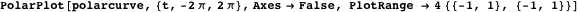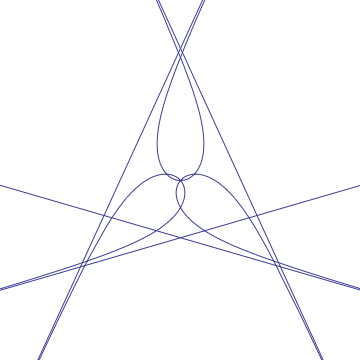The default view point shows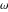, the image of the point at infinity.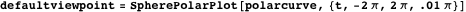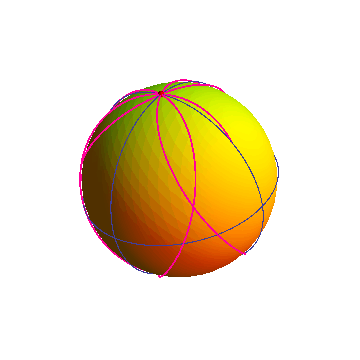Here is an animation where the view point goes once around the equator. The blue point zero is the image of the origin in the plane.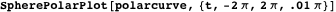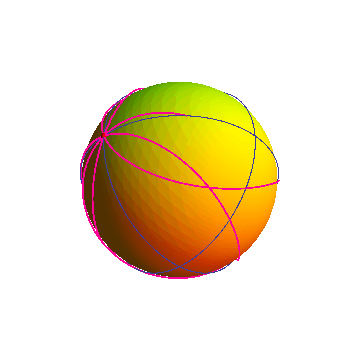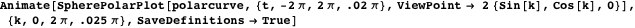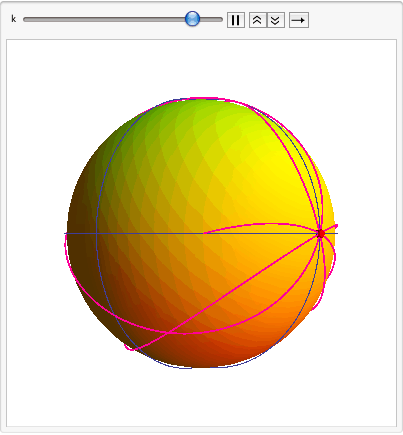To see through the sphere we use the Opacity option.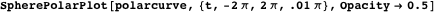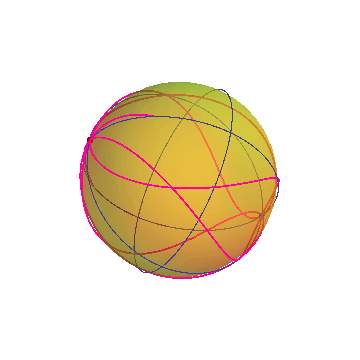#### Sensitivity and Faithfulness

This modified inverse stereographic projectionis very sensitive close to the origin: two points near the origin in the plane will appear far apart on the sphere. On the other hand, points near infinity will appear close together, thus showing the asymptotic behavior that is the qualitative property of the curve far away from the origin.

Faithfulness means that mapping the plane curve to the sphere does not alter the shape of the curve. In particular, at, the slopes of the different branches are exactly what they should be. We illustrate this by plotting the function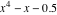.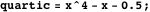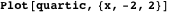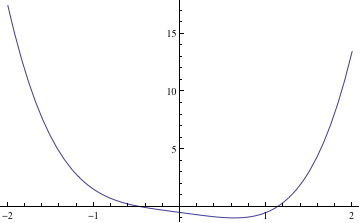Making the interval somewhat larger loses details close to the origin.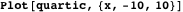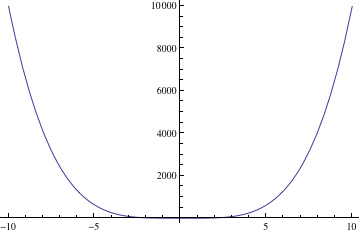Here is the same curve on the sphere, viewed to show zero and then.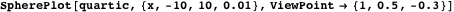The derivatives at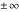are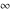, and, indeed, we see that the curve is vertical near.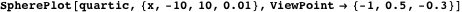#### Unveiling the True Nature of a Curve

Geometers know that plane geometry is incomplete. We have to look at a curve in the projective plane (i.e., the Riemann sphere) to get complete results.

For example, students in high school are told that hyperbolas have two branches.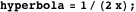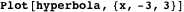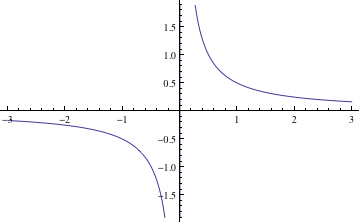In this animation, a hyperbola appears as a one-branched figure-eight curve on the sphere.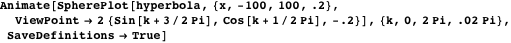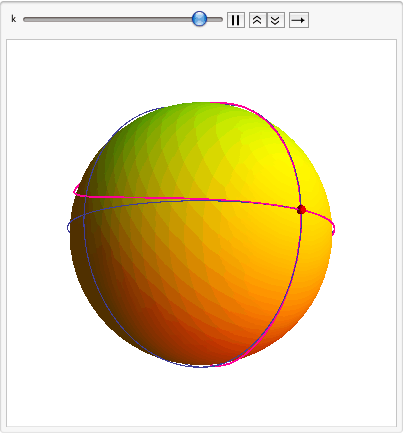### The Mapping

We define the mapping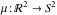using the following picture.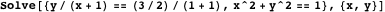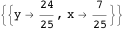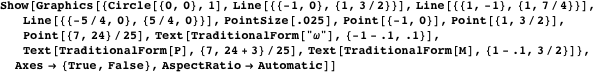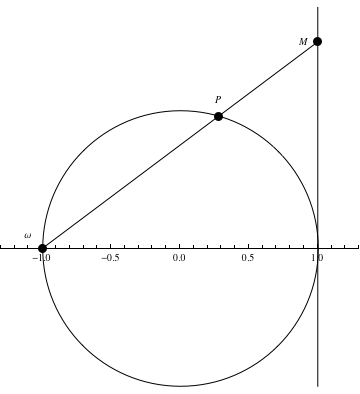The projection pole is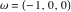,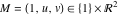and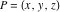is the point corresponding to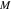on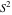. Then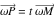and to find the mapping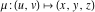, we solve for.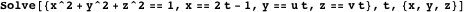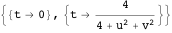One solution gives the pointand the other solution determines the mapping to the sphere.

### The Program: Parametric Curves on the Sphere

The modified inverse stereographic functionturns out to be fairly simple.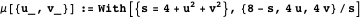We define the sphere and the equator, two meridians, a blue zero, and a redfor orientation.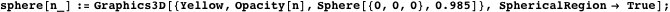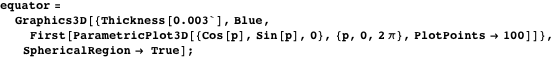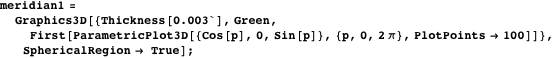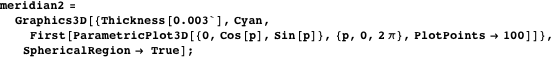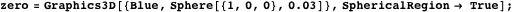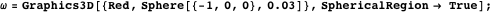To avoid infinities, SphereParametricPlot is based on ScatterPlot3D rather than ParametricPlot3D. In analogy with Plot and PolarPlot, we define the functions SpherePlot and SpherePolarPlot, both in terms of SphereParametricPlot.

The first argument to SphereParametricPlot is a pair of functions. The first argument to SpherePlot or SpherePolarPlot is a single function. The second argument to all three functions is a range specification. A ViewPoint option may be given.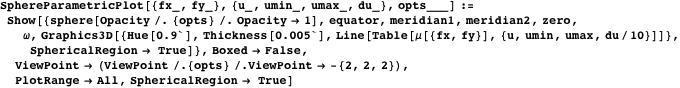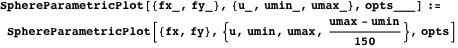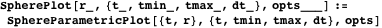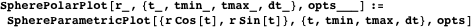Here is one final example.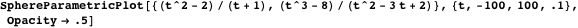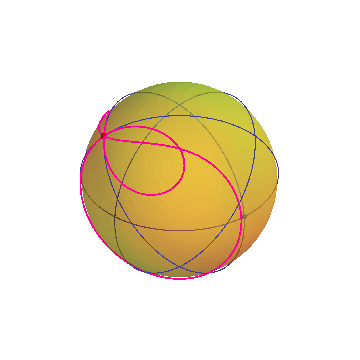ImplicitEquOnSphere.nb

### References

 D. Benayat, “Graphing on the Riemann Sphere,” The Mathematica Journal, 2011. dx.doi.org/doi:10.3888/tmj.10.4-5.

Djilali Benayat received a B.Sc. in mathematics (1968) and a Diploma of Advanced Studies (DEA) in functional analysis (1969) from Algiers University, a DEA in algebraic topology (1970) and a “Doctorat de 3e cycle” in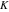-theory (1972) from Strasbourg University, and a D.Sc. in algebraic topology (Theory of Adams Algebras in Stable Homotopy) (1987) from Metz and Algiers universities. Presently he is a professor of mathematics at the École Normale Supérieure of Algiers, Algeria. He has supervised a master’s thesis and three D.Sc.’s in algebraic topology and number theory.